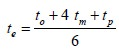Home | ARTS | Operations Management | Introduction of Pert

# Introduction of Pert

Posted On :  23.06.2018 11:01 pm

Programme Evaluation and Review Technique (PERT) is a tool that would help a project manager in project planning and control.

Introduction

Programme Evaluation and Review Technique (PERT) is a tool that would help a project manager in project planning and control. It would enable him in continuously monitoring a project and taking corrective measures wherever necessary. This technique involves statistical methods.

## Assumptions for Pert

Note that in CPM, the assumption is that precise time estimate is available for each activity in a project. However, one finds most of the times that this is not practically possible.

In PERT, we assume that it is not possible to have precise time estimate for each activity and instead, probabilistic estimates of time alone are possible. A multiple time estimate approach is followed here. In probabilistic time estimate, the following 3 types of estimate are possible:

Pessimistic time estimate ( tp  )

Optimistic time estimate ( to  )

Most likely time estimate ( tm  )

The optimistic estimate of time is based on the assumption that an activity will not involve any difficulty during execution and it can be completed within a short period. On the other hand, a pessimistic estimate is made on the assumption that there would be unexpected problems during the execution of an activity and hence it would consume more time. The most likely time estimate is made in between the optimistic and the pessimistic estimates of time. Thus the three estimates of time have the relationship

t o t m tp

Practically speaking, neither the pessimistic nor the optimistic estimate may hold in reality and it is the most likely time estimate that is expected to prevail in almost all cases. Therefore, it is preferable to give more weight to the most likely time estimate.

We give a weight of 4 to most likely time estimate and a weight of 1 each to the pessimistic and optimistic time estimates. We arrive at a time estimate ( te ) as the weighted average of these estimates as follows:Since we have taken 6 units ( 1 for tp , 4 for tm and 1 for to ), we divide the sum by 6. With this time estimate, we can determine the project completion time as applicable for CPM.

Since PERT involves the average of three estimates of time for each activity, this method is very practical and the results from PERT will be have a reasonable amount of reliability.
Tags : Operations Management - Network Problems
Last 30 days 6480 views